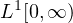Electron. J. Differential Equations, Vol. 2021 (2021), No. 80, pp. 1-22.

### A fractional Gronwall inequality and the asymptotic behaviour of global solutions of Caputo fractional problems Jeffrey R. L. Webb

Abstract:
We study the asymptotic behaviour of global solutions of some nonlinear integral equations related to some Caputo fractional initial value problems. We consider problems of fractional order between 0 and 1 and of order between 1 and 2, each in two cases: when the nonlinearity depends only on the function, and when the nonlinearity also depends on fractional derivatives of lower order. Our main tool is a new Gronwall inequality for integrals with singular kernels, which we prove here, and a related boundedness property of a fractional integral of anfunction.

Submitted July 23, 2021. Published September 20, 2021.
Math Subject Classifications: 34A08, 34A12, 26A33, 26D10.
Key Words: Fractional derivatives; asymptotic behaviour; Gronwall inequality; weakly singular kernel.
DOI: https://doi.org/10.58997/ejde.2021.80

Show me the PDF file (384 KB), TEX file for this article.Jeffrey R. L. Webb School of Mathematics and Statistics University of Glasgow Glasgow G12 8SQ, UK email: jeffrey.webb@glasgow.ac.uk